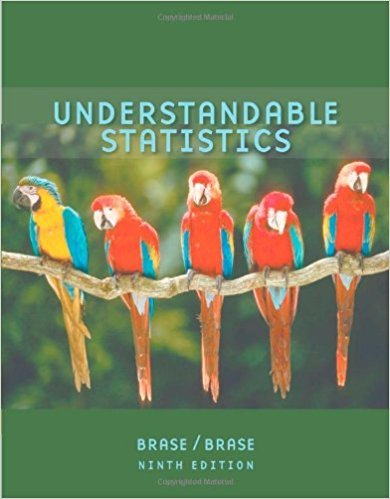×
×

# Solutions for Chapter 6.2: NORMAL DISTRIBUTIONS## Full solutions for Understandable Statistics | 9th Edition

ISBN: 9780618949922Solutions for Chapter 6.2: NORMAL DISTRIBUTIONS

Solutions for Chapter 6.2
4 5 0 399 Reviews
31
5
##### ISBN: 9780618949922

This expansive textbook survival guide covers the following chapters and their solutions. Understandable Statistics was written by and is associated to the ISBN: 9780618949922. Since 48 problems in chapter 6.2: NORMAL DISTRIBUTIONS have been answered, more than 38138 students have viewed full step-by-step solutions from this chapter. This textbook survival guide was created for the textbook: Understandable Statistics, edition: 9. Chapter 6.2: NORMAL DISTRIBUTIONS includes 48 full step-by-step solutions.

Key Statistics Terms and definitions covered in this textbook
• Additivity property of x 2

If two independent random variables X1 and X2 are distributed as chi-square with v1 and v2 degrees of freedom, respectively, Y = + X X 1 2 is a chi-square random variable with u = + v v 1 2 degrees of freedom. This generalizes to any number of independent chi-square random variables.

• Alternative hypothesis

In statistical hypothesis testing, this is a hypothesis other than the one that is being tested. The alternative hypothesis contains feasible conditions, whereas the null hypothesis speciies conditions that are under test

• Analytic study

A study in which a sample from a population is used to make inference to a future population. Stability needs to be assumed. See Enumerative study

• Bias

An effect that systematically distorts a statistical result or estimate, preventing it from representing the true quantity of interest.

• Central limit theorem

The simplest form of the central limit theorem states that the sum of n independently distributed random variables will tend to be normally distributed as n becomes large. It is a necessary and suficient condition that none of the variances of the individual random variables are large in comparison to their sum. There are more general forms of the central theorem that allow ininite variances and correlated random variables, and there is a multivariate version of the theorem.

• Coeficient of determination

See R 2 .

• Confounding

When a factorial experiment is run in blocks and the blocks are too small to contain a complete replicate of the experiment, one can run a fraction of the replicate in each block, but this results in losing information on some effects. These effects are linked with or confounded with the blocks. In general, when two factors are varied such that their individual effects cannot be determined separately, their effects are said to be confounded.

• Conidence coeficient

The probability 1?a associated with a conidence interval expressing the probability that the stated interval will contain the true parameter value.

• Continuous uniform random variable

A continuous random variable with range of a inite interval and a constant probability density function.

• Control limits

See Control chart.

• Correlation matrix

A square matrix that contains the correlations among a set of random variables, say, XX X 1 2 k , ,…, . The main diagonal elements of the matrix are unity and the off-diagonal elements rij are the correlations between Xi and Xj .

• Crossed factors

Another name for factors that are arranged in a factorial experiment.

• Curvilinear regression

An expression sometimes used for nonlinear regression models or polynomial regression models.

• Deming

W. Edwards Deming (1900–1993) was a leader in the use of statistical quality control.

• Deming’s 14 points.

A management philosophy promoted by W. Edwards Deming that emphasizes the importance of change and quality

• Discrete random variable

A random variable with a inite (or countably ininite) range.

• Erlang random variable

A continuous random variable that is the sum of a ixed number of independent, exponential random variables.

• Error of estimation

The difference between an estimated value and the true value.

• Estimator (or point estimator)

A procedure for producing an estimate of a parameter of interest. An estimator is usually a function of only sample data values, and when these data values are available, it results in an estimate of the parameter of interest.

• Goodness of fit

In general, the agreement of a set of observed values and a set of theoretical values that depend on some hypothesis. The term is often used in itting a theoretical distribution to a set of observations.

×